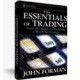Categories

# The Primary Trade Size Forumla

Position sizing is something that comes up ALL THE TIME in the discussions among new and developing traders. Everything starts with the size of the risk we’re looking to take and works up from there.

Here is the basic formula:

Position Size = Amount at risk /(Number of Points or Pips being risked x Value of  Each Point or Pip)

Amount at risk comes from looking at the fraction of your account that you want to be exposed to loss on the given trade. So if you have a \$5000 account and want to risk 2% then that would be \$100.

The number of points or pips being risked is basically how far away from your entry point your initial stop will be. If you’re getting long at 100 and your stop is at 95, then the point risk is 5.

The value of each point or pip will depend on the market you’re trading.

Thinking in stock market terms where the value of a point is \$1.00 per share (adjust for your own currency), the formula would be as follows:

Shares = \$ to be risked / (Points risked)

So if you want to risk \$1000 and your point risk is 10 you would trade 100 shares: \$1000/(10 x \$1)

If you’re trading a fixed size contract market such as futures or forex (lots), then it would look like this:

Contracts (Lots) = \$ to be risk / (points risked x point value)

In the case of e-mini S&P 500 futures, the point value is \$50, so if we want to risk \$1000 and have a 10 point stop, we would trade 2 contracts: \$1000/(10 x \$50)

If we are trading micro lots of EUR/USD where the pip value is \$0.10 and we are risking \$1000 with a 125 point stop, we’d trade 80 contracts: \$1000/(125 x \$0.10)

Notice that nowhere in here did I bring up the question of appropriate leverage. It only matters if you don’t have enough available to you to put on the trade you’re looking to do.## By John

Author of The Essentials of Trading